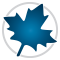Statistics Education - Maple Features - Maplesoft# Maple

Statistics Education

Maple provides a rich environment for statistics education, helping teachers present and students understand a wide variety of topics from probability and statistics.

• Explore descriptive statistics, probability calculations, simulation, estimation, testing, and visualization.
• Focus on the concepts, not the mechanics of the calculations, with Clickable Statistics tools that support exploration and interactive learning.
• Context-sensitive menus allow students to easily perform a wide variety of statistics computations and visualizations with the click of a mouse
• Tutors guide students through important concepts, such as exploring properties of distributions and choosing an appropriate hypothesis test
• Math Apps provide interactive, highly visual explorations of key ideas from statistics, reinforcing and deepening student understanding
• Easily create custom interactive applications that deepen understanding, such as allowing students to interactively resample data and immediately see the results.
• Perform advanced statistics and data analysis, such as curve fitting, regression analysis, time series analysis, financial analysis, and much more.
• Leverage a symbolic computation framework that allows students to work directly with the same mathematical expressions found in their textbooks, so they can focus on developing their understanding of the theory.  For example:
• Define custom distributions by specifying a mathematical formula for the probability function or expressing the mathematical relationship between existing continuous or discrete distributions
• Use these custom distributions in any computation or visualization where a built-in distribution can be used
• Rely on arbitrary precision numeric calculations for accurate results.
• Take advantage of everything the Maple environment has to offer while teaching and learning statistics, including:
• Data import and export
• Standard math notation
• Extensive document creation and word-processing tools
• Sophisticated programming language designed for mathematical computations

### Maple T.A. is ideal for statistics!

Hundreds of free statistics questions are available for automated testing and assessment.

Video Tutorials: Explore Statistics With Maple
In the videos below, see how Maple can be used to explore a variety of topics in statistics education.

Descriptive Statistics
Inferential Statistics

Random Variables and Probability Distributions## Prêt(e) pour l'étape suivante ?

*Ce programme d'évaluation n'est proposé actuellement ni aux étudiants ni aux particuliers.

Achetez: